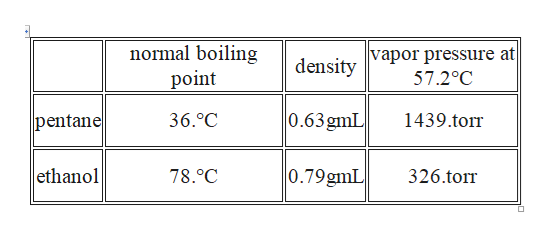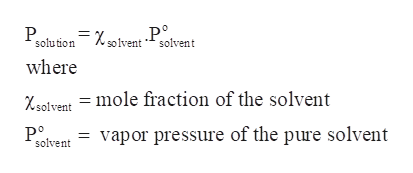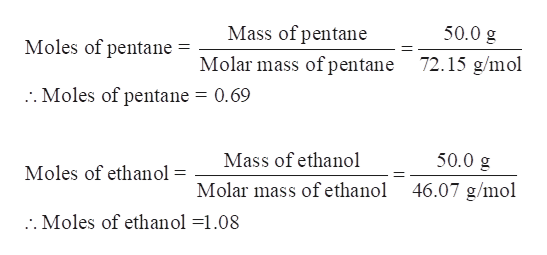# A solution of pentane and ethanol (CH3CH2OH) that is 50.% pentane by mass is boiling at 57.2°C. The vapor is collected and cooled until it condenses to form a new solution. Calculate the percent by mass of pentane in the new solution. Here's some data you may need: normal boiling pointdensityvapor pressure at 57.2°Cpentane36.°C0.63gmL1439.torrethanol78.°C0.79gmL326.torrBe sure your answer has 2 significant digits.dont round during math only for answer!Note for advanced students: you may assume the solution and vapor above it are ideal.

Question
24 views

A solution of pentane and ethanol (CH3CH2OH)

that is 50.% pentane by mass is boiling at 57.2°C. The vapor is collected and cooled until it condenses to form a new solution.

Calculate the percent by mass of pentane in the new solution. Here's some data you may need:

 normal boiling point density vapor pressure at  57.2°C pentane 36.°C 0.63gmL 1439.torr ethanol 78.°C 0.79gmL 326.torr

dont round during math only for answer!

Note for advanced students: you may assume the solution and vapor above it are ideal.

check_circle

Step 1

A solution of pentane and ethanol (CH3CH2OH) that is 50.0 % pentane by mass is boiling at 57.2°C. The vapor is collected and cooled until it condenses to form a new solution. The percent by mass of pentane in the new solution is to be calculated.help_outlineImage Transcriptionclosenormal boiling vapor pressure at 57.2°C density point 0.63gmL pentane 36.°C 1439.tor ethanol 0.79gmL 78.0C 326.torr fullscreen
Step 2

When two solutions are mixed, then the total vapor pressure is equal to the sum of the partial pressure of each solution i.e.

PTotal = Psolution 1 + Psolution 2

And according to Raoult’s Law, the vapor pressure of the solution is equal to the vapor pressure of the pure solvent at same temperature multiplied by the mole fraction of the solvent.help_outlineImage TranscriptioncloseР Р°. solvent *- solvent solution where Xsolvent = mole fraction of the solvent Pе solvent vapor pressure of the pure solvent fullscreen
Step 3

Calculate the moles of pentane and ethanol in the given solution.

The given solution is 50.0 % pentane by mass which means in 100g of solution:

Mass of pen...help_outlineImage TranscriptioncloseMass of pentane 50.0 g Moles of pentane Molar mass of pentane 72.15 g/mol .. Moles of pentane = 0.69 Mass of ethanol 50.0 g Moles of ethanol Molar mass of ethanol 46.07 g/mol . Moles of ethanol =1.08 fullscreen

### Want to see the full answer?

See Solution

#### Want to see this answer and more?

Solutions are written by subject experts who are available 24/7. Questions are typically answered within 1 hour.*

See Solution
*Response times may vary by subject and question.
Tagged in

### Chemistry